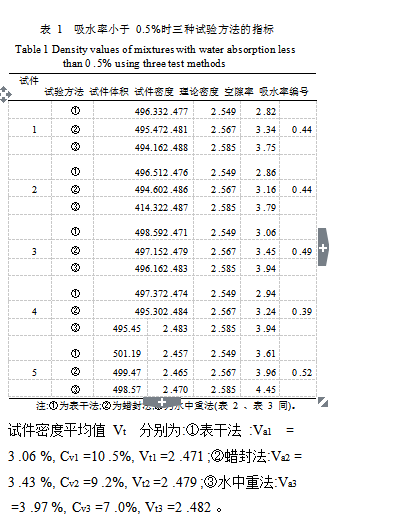# 压实沥青混合料密度试验方法分析

1 表干法的适用条件及影响因素

2 水中重法的适用条件分析

3 蜡封法的适用条件分析

4 体积法的适用条件及影响因素分析

5 表干法、水中重法和蜡封法试验结果分析比较

5 .1  吸水率小于0 .5%时的试验结果分析5 .2  吸水率为 0 .5%～ 2 .0%时的试验结果分析

4 .45 %, Cv1  =10 .0%, Vt1  =2 .429 ;蜡封法 :Va2 =

4 .87 %, Cv2 =3 .2%, Vt2 =2 .434 ;水中重法 :Va3 =

4 .45 %, Cv3 =4 .6%, Vt3 =2 .460 ;从上述统计指标

5 .3  吸水率大于 2 .0%时的试验结果分析

6 .51 %, Cv1 =10 .3%, Vt1 =2 .373 ;②蜡封法:Va2 =

6 .21 %, Cv2 =4 .9%, Vt2 =2 .395 ;③水中重法:Va3

=5 .52 %, Cv3 =11 .9%, Vt3 =2 .427 ;从上述统计结

6 结语